Hits: 2

# Deletion From a Red-Black Tree

#### In this tutorial, you will learn how a node is deleted from a red-black tree is. Also, you will find working examples of deletions performed on a red-black tree in Python.

Red-Black tree is a self-balancing binary search tree in which each node contains an extra bit for denoting the color of the node, either red or black.

Before reading this article, please refer to the article on red-black tree.

Deleting a node may or may not disrupt the red-black properties of a red-black tree. If this action violates the red-black properties, then a fixing algorithm is used to regain the red-black properties.

## Deleting an element from a Red-Black Tree

This operation removes a node from the tree. After deleting a node, the red-black property is maintained again.

1. Let the `nodeToBeDeleted` be: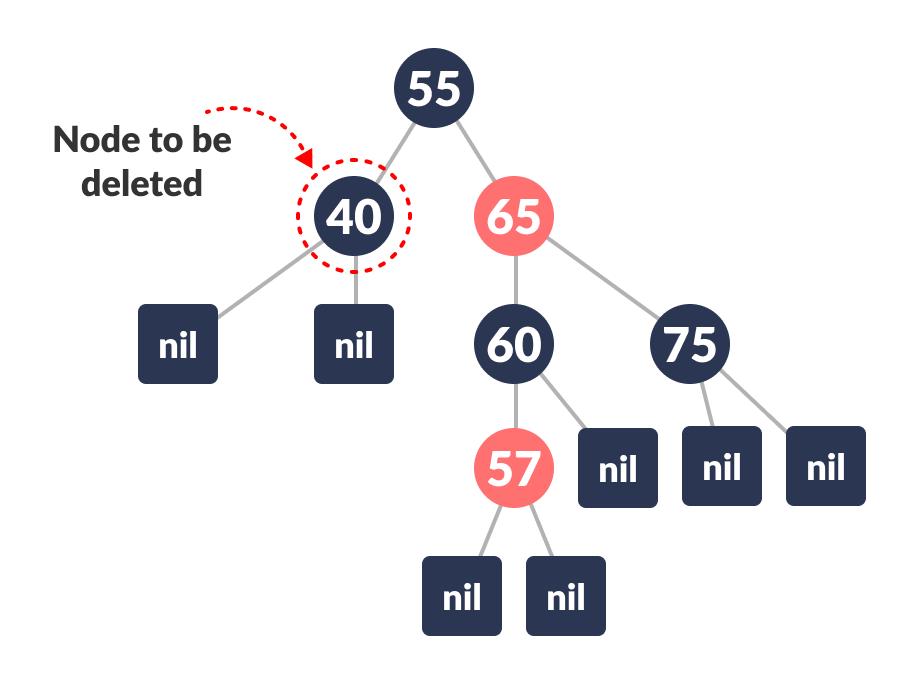Node to be deleted
2. Save the color of nodeToBeDeleted in origrinalColor.Saving original color
3. If the left child of nodeToBeDeleted is `NULL`
1. Assign the right child of nodeToBeDeleted to x.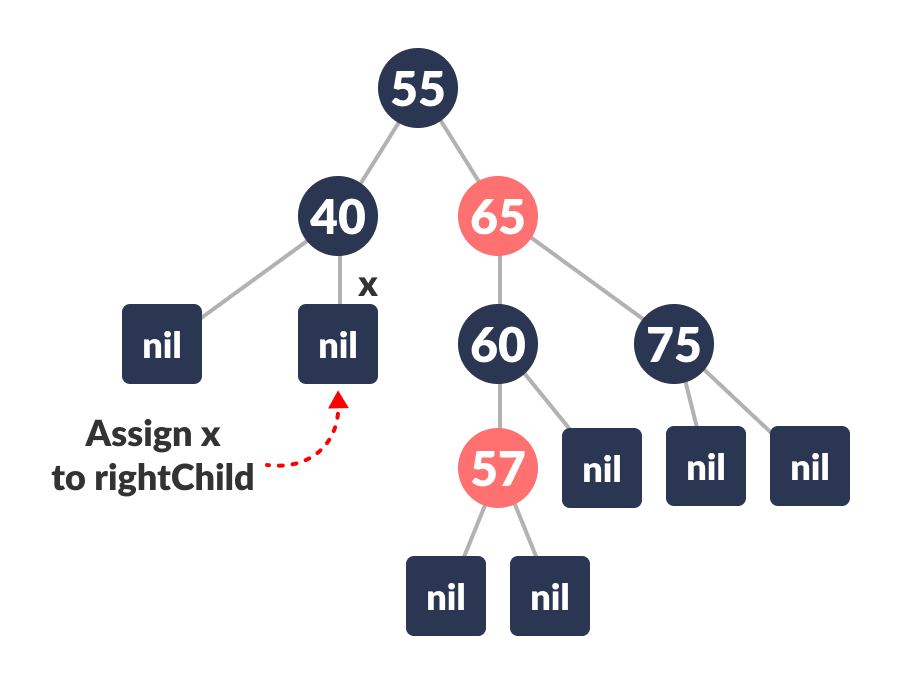Assign x to the rightChild
2. Transplant nodeToBeDeleted with x.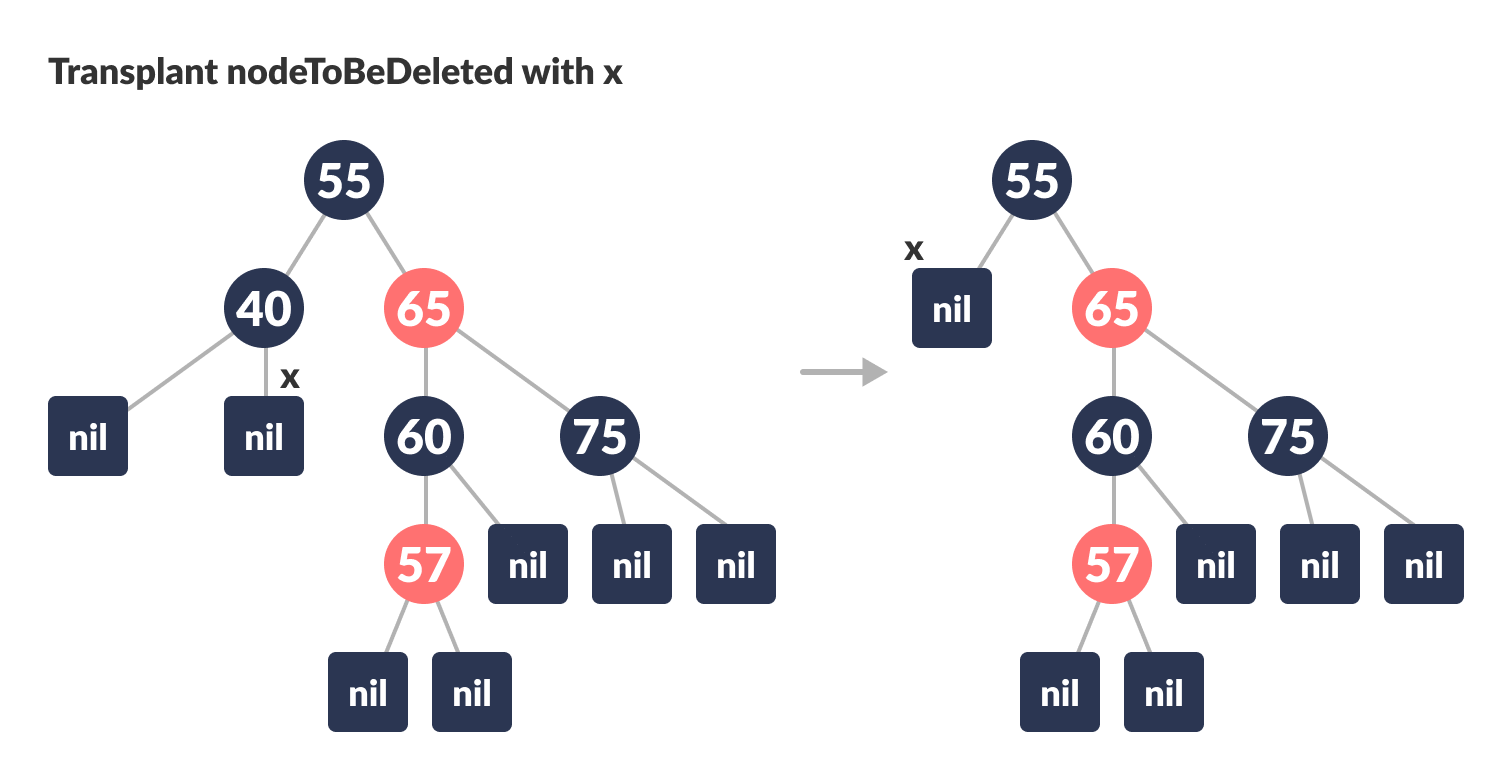Transplant nodeToBeDeleted with x
4. Else if the right child of nodeToBeDeleted is `NULL`
1. Assign the left child of nodeToBeDeleted into x.
2. Transplant nodeToBeDeleted with x.
5. Else
1. Assign the minimum of right subtree of noteToBeDeleted into y.
2. Save the color of y in originalColor.
3. Assign the rightChild of y into x.
4. If y is a child of nodeToBeDeleted, then set the parent of x as y.
5. Else, transplant y with rightChild of y.
6. Transplant nodeToBeDeleted with y.
7. Set the color of y with originalColor.
6. If the originalColor is BLACK, call DeleteFix(x).

## Algorithm to maintain Red-Black property after deletion

This algorithm is implemented when a black node is deleted because it violates the black depth property of the red-black tree.

This violation is corrected by assuming that node x (which is occupying y‘s original position) has an extra black. This makes node x neither red nor black. It is either doubly black or black-and-red. This violates the red-black properties.

However, the color attribute of x is not changed rather the extra black is represented in x‘s pointing to the node.

The extra black can be removed if

1. It reaches the root node.
2. If x points to a red-black node. In this case, x is colored black.
3. Suitable rotations and recolorings are performed.

Following algorithm retains the properties of a red-black tree.

1. Do the following until the x is not the root of the tree and the color of x is BLACK
2. If x is the left child of its parent then,
1. Assign `w` to the sibling of `x`.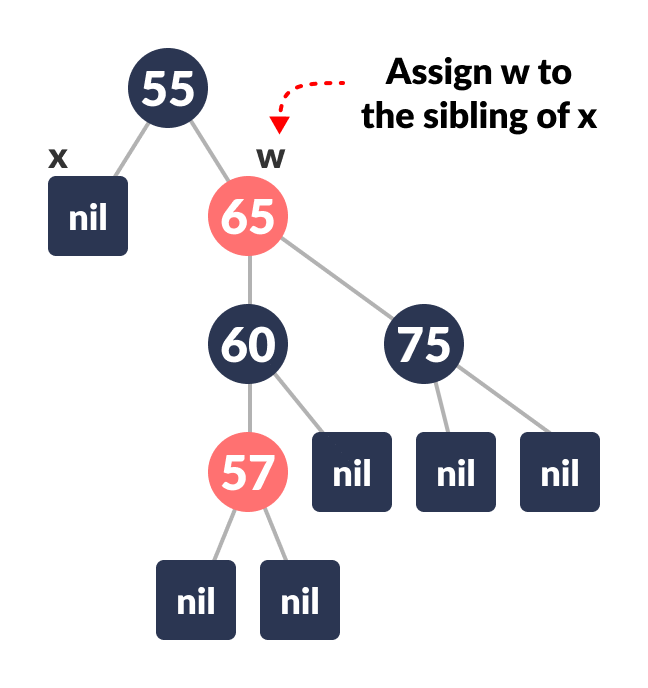Assigning w
2. If the sibling of x is RED,
Case-I:

1. Set the color of the right child of the parent of x as BLACK.
2. Set the color of the parent of x as RED.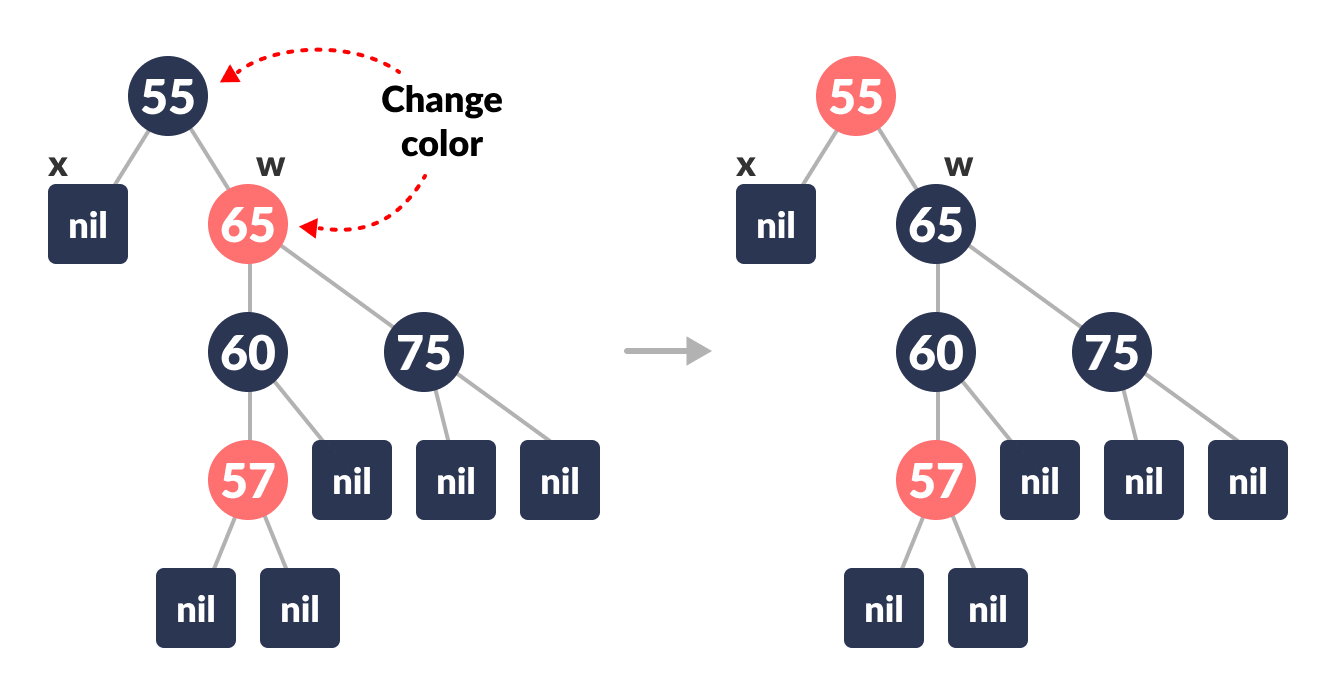Color change
3. Left-Rotate the parent of x.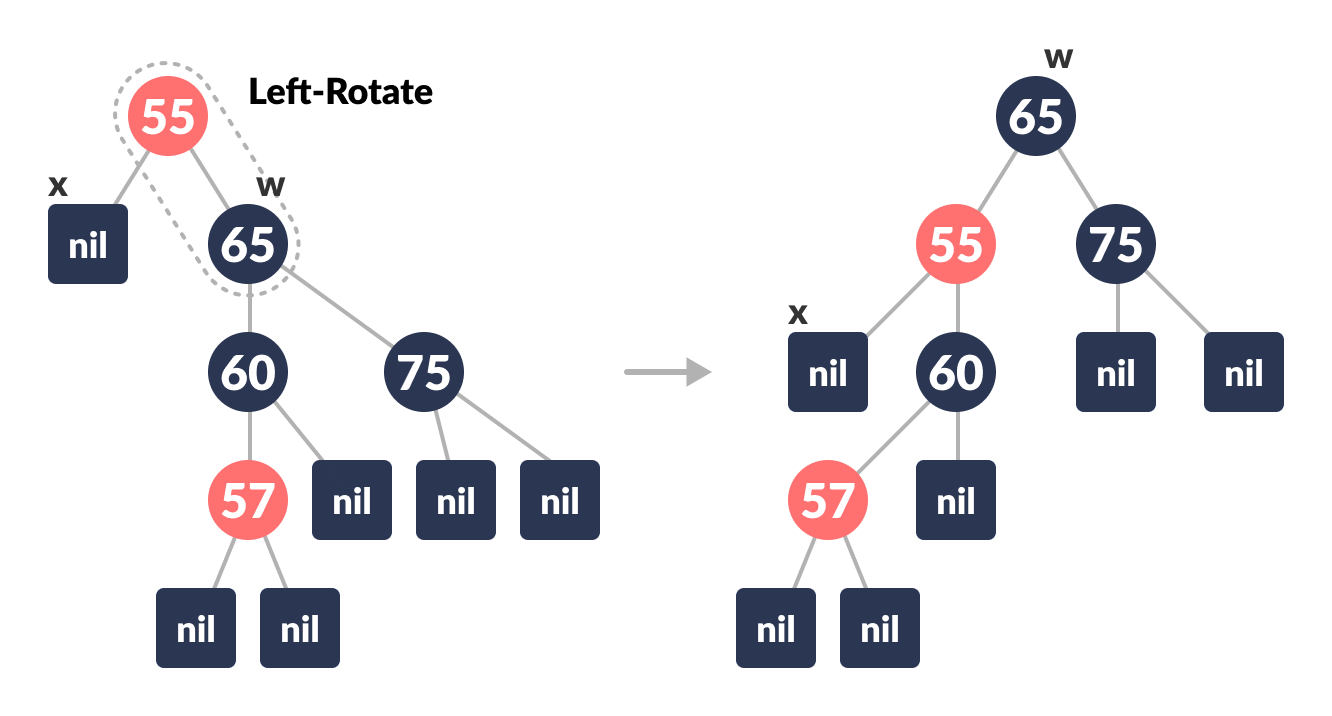Left-rotate
4. Assign the rightChild of the parent of x to w.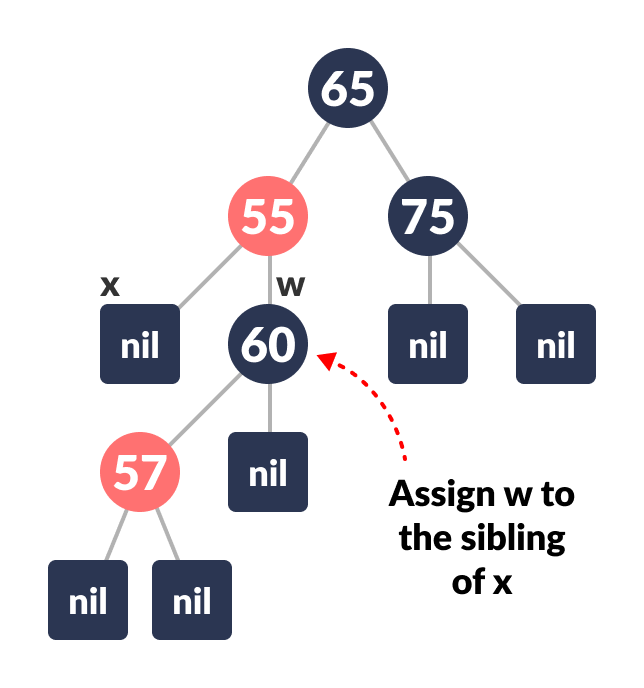Reassign w
3. If the color of both the right and the leftChild of w is BLACK,
Case-II:

1. Set the color of w as RED
2. Assign the parent of x to x.
4. Else if the color of the rightChild of w is BLACK
Case-III:

1. Set the color of the leftChild of w as BLACK
2. Set the color of w as RED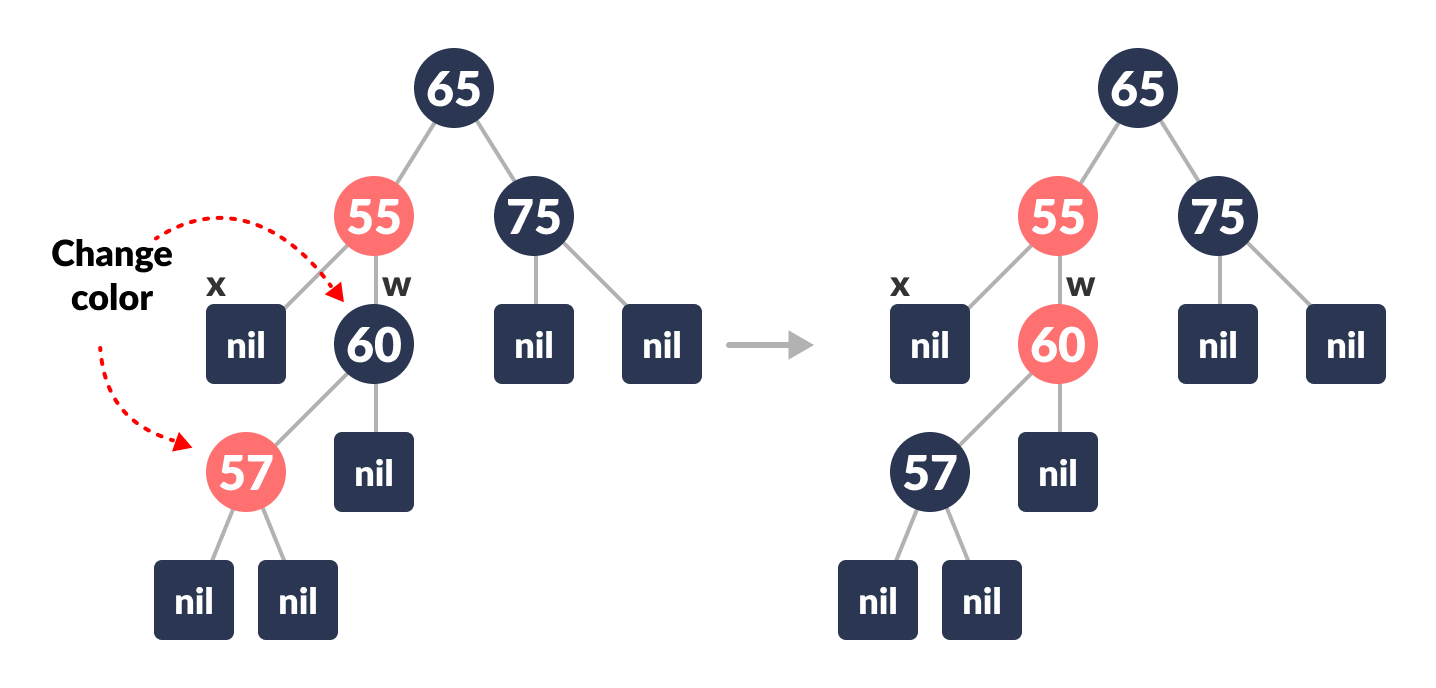Color change
3. Right-Rotate w.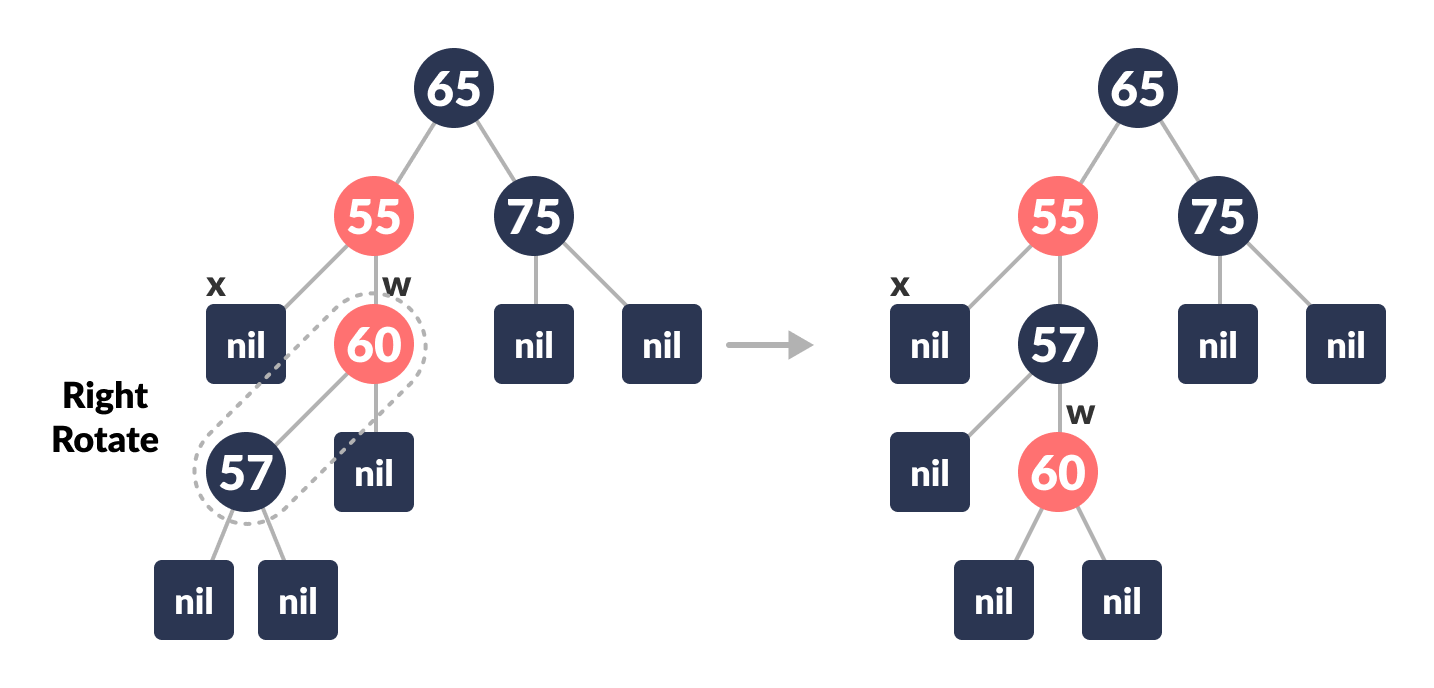Right rotate
4. Assign the rightChild of the parent of x to w.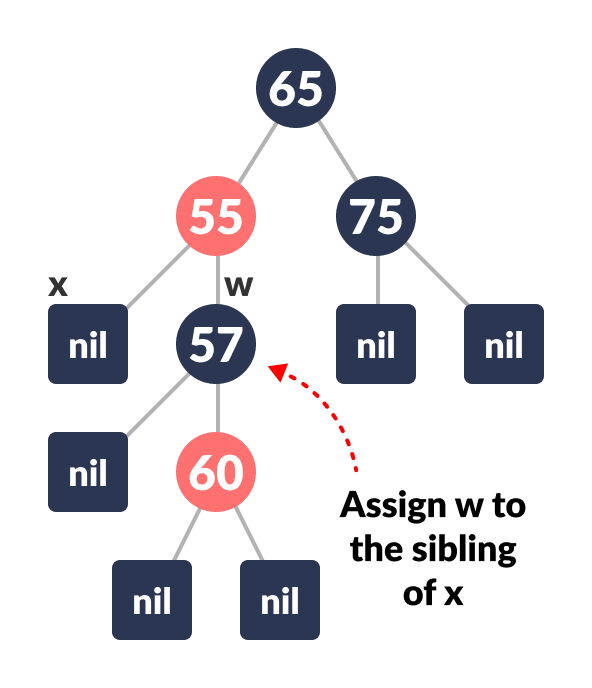Reassign w
5. If any of the above cases do not occur, then do the following.
Case-IV:

1. Set the color of w as the color of the parent of x.
2. Set the color of the parent of parent of x as BLACK.
3. Set the color of the right child of w as BLACK.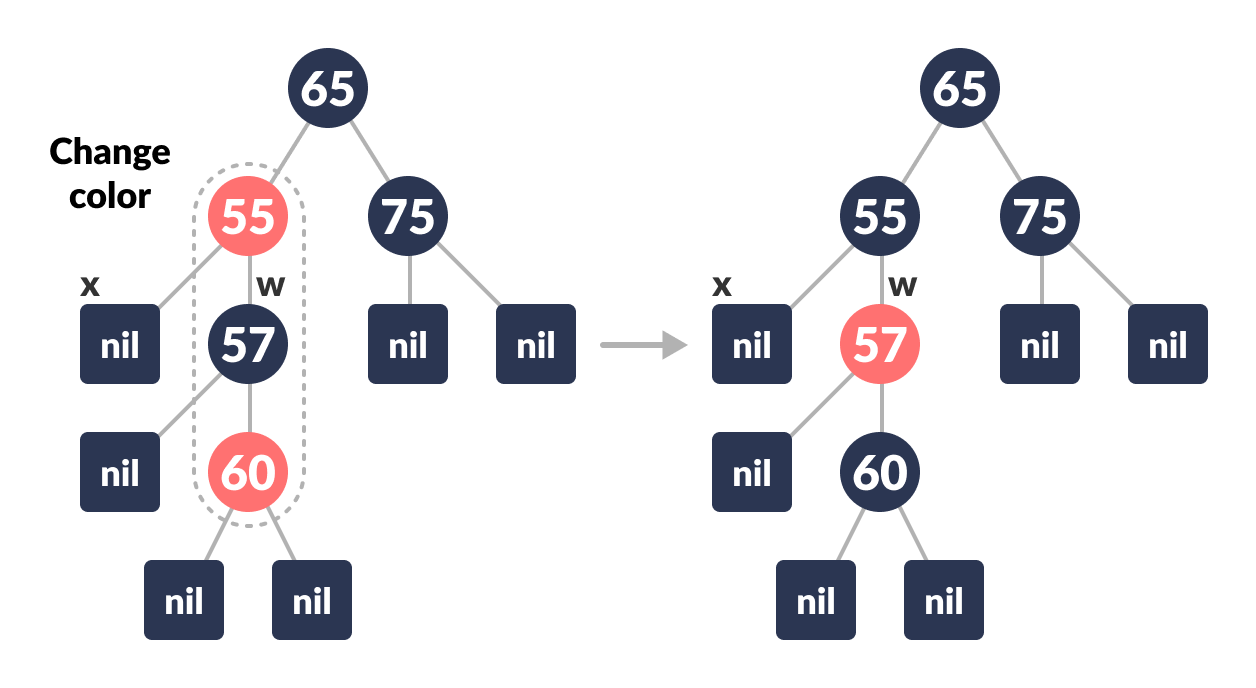Color change
4. Left-Rotate the parent of x.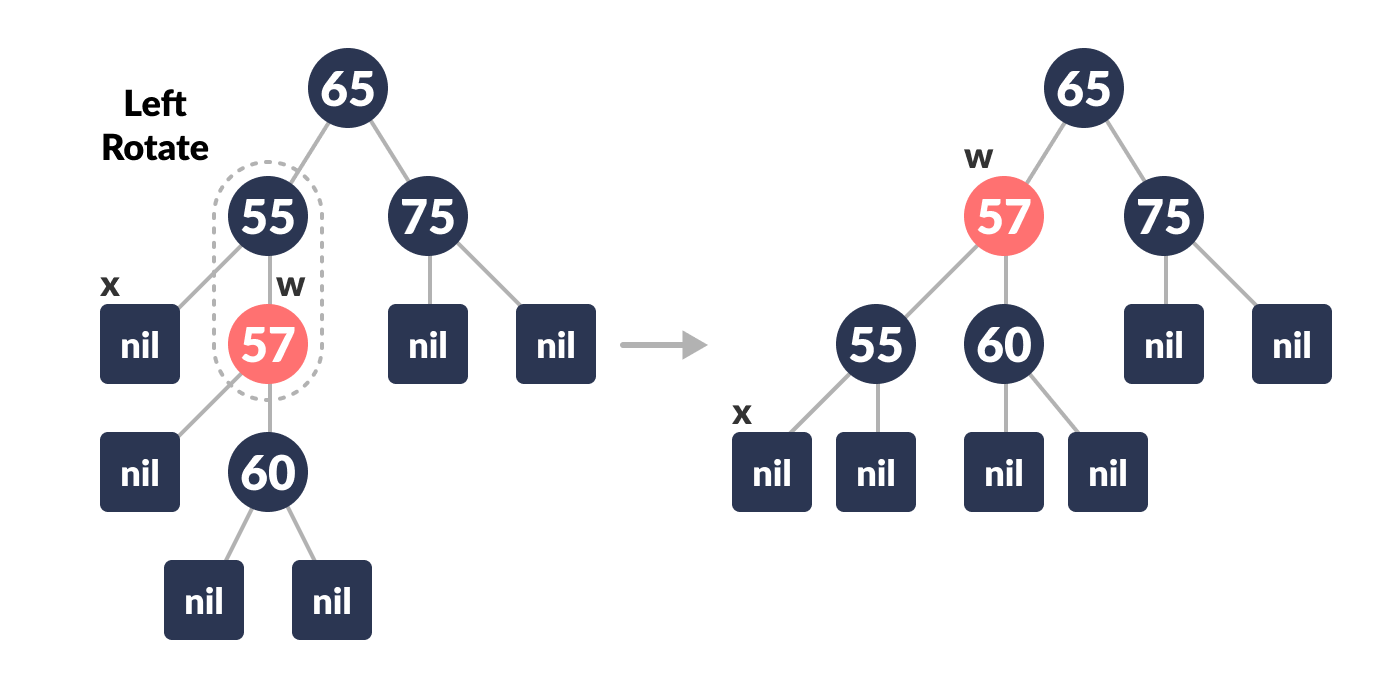Left-rotate
5. Set x as the root of the tree.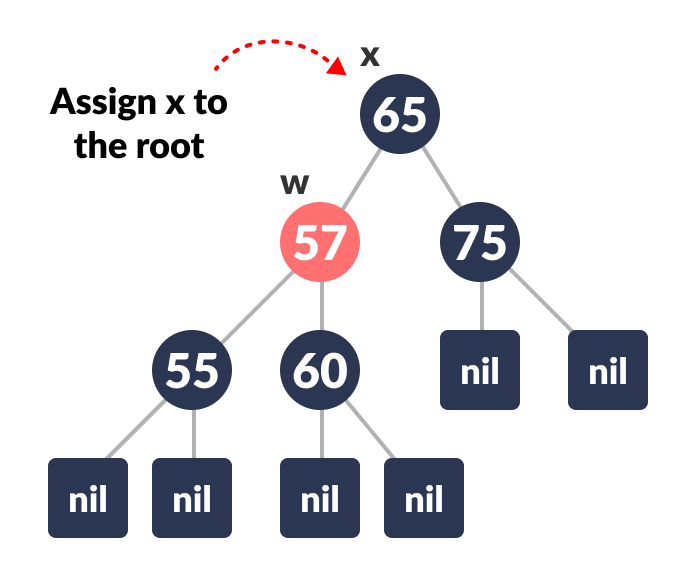Set x as root
3. Else same as above with right changed to left and vice versa.
4. Set the color of x as BLACK.

The workflow of the above cases can be understood with the help of the flowchart below.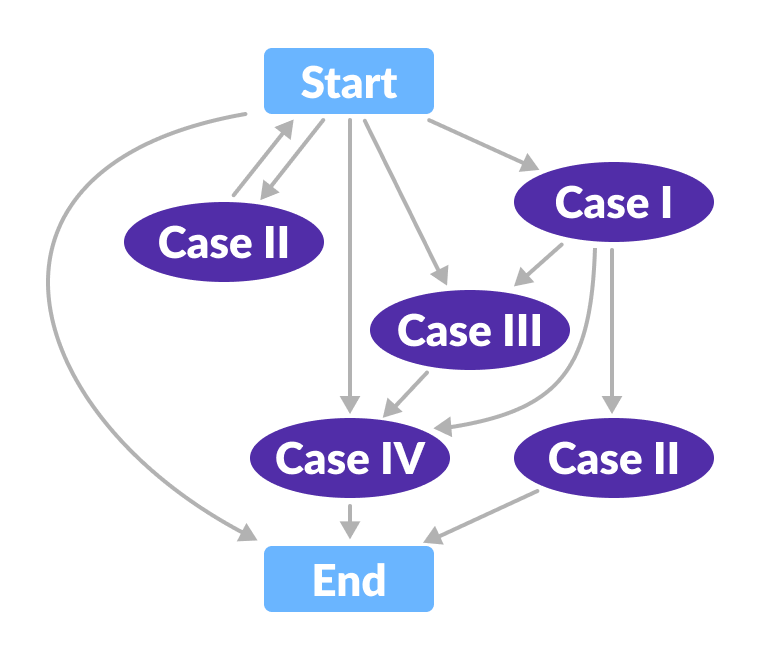Flowchart for deletion operation

## Python Examples

``````/* Implementing Red-Black Tree in Python */

import sys

/* Node creation */
class Node():
def __init__(self, item):
self.item = item
self.parent = None
self.left = None
self.right = None
self.color = 1

class RedBlackTree():
def __init__(self):
self.TNULL = Node(0)
self.TNULL.color = 0
self.TNULL.left = None
self.TNULL.right = None
self.root = self.TNULL

/* Preorder */
def pre_order_helper(self, node):
if node != TNULL:
sys.stdout.write(node.item + " ")
self.pre_order_helper(node.left)
self.pre_order_helper(node.right)

/* Inorder */
def in_order_helper(self, node):
if node != TNULL:
self.in_order_helper(node.left)
sys.stdout.write(node.item + " ")
self.in_order_helper(node.right)

/* Postorder */
def post_order_helper(self, node):
if node != TNULL:
self.post_order_helper(node.left)
self.post_order_helper(node.right)
sys.stdout.write(node.item + " ")

/* Search the tree */
def search_tree_helper(self, node, key):
if node == TNULL or key == node.item:
return node

if key < node.item:
return self.search_tree_helper(node.left, key)
return self.search_tree_helper(node.right, key)

/* Balancing the tree after deletion */
def delete_fix(self, x):
while x != self.root and x.color == 0:
if x == x.parent.left:
s = x.parent.right
if s.color == 1:
s.color = 0
x.parent.color = 1
self.left_rotate(x.parent)
s = x.parent.right

if s.left.color == 0 and s.right.color == 0:
s.color = 1
x = x.parent
else:
if s.right.color == 0:
s.left.color = 0
s.color = 1
self.right_rotate(s)
s = x.parent.right

s.color = x.parent.color
x.parent.color = 0
s.right.color = 0
self.left_rotate(x.parent)
x = self.root
else:
s = x.parent.left
if s.color == 1:
s.color = 0
x.parent.color = 1
self.right_rotate(x.parent)
s = x.parent.left

if s.right.color == 0 and s.right.color == 0:
s.color = 1
x = x.parent
else:
if s.left.color == 0:
s.right.color = 0
s.color = 1
self.left_rotate(s)
s = x.parent.left

s.color = x.parent.color
x.parent.color = 0
s.left.color = 0
self.right_rotate(x.parent)
x = self.root
x.color = 0

def __rb_transplant(self, u, v):
if u.parent == None:
self.root = v
elif u == u.parent.left:
u.parent.left = v
else:
u.parent.right = v
v.parent = u.parent

/* Node deletion */
def delete_node_helper(self, node, key):
z = self.TNULL
while node != self.TNULL:
if node.item == key:
z = node

if node.item <= key:
node = node.right
else:
node = node.left

if z == self.TNULL:
print("Cannot find key in the tree")
return

y = z
y_original_color = y.color
if z.left == self.TNULL:
x = z.right
self.__rb_transplant(z, z.right)
elif (z.right == self.TNULL):
x = z.left
self.__rb_transplant(z, z.left)
else:
y = self.minimum(z.right)
y_original_color = y.color
x = y.right
if y.parent == z:
x.parent = y
else:
self.__rb_transplant(y, y.right)
y.right = z.right
y.right.parent = y

self.__rb_transplant(z, y)
y.left = z.left
y.left.parent = y
y.color = z.color
if y_original_color == 0:
self.delete_fix(x)

/* Balance the tree after insertion */
def fix_insert(self, k):
while k.parent.color == 1:
if k.parent == k.parent.parent.right:
u = k.parent.parent.left
if u.color == 1:
u.color = 0
k.parent.color = 0
k.parent.parent.color = 1
k = k.parent.parent
else:
if k == k.parent.left:
k = k.parent
self.right_rotate(k)
k.parent.color = 0
k.parent.parent.color = 1
self.left_rotate(k.parent.parent)
else:
u = k.parent.parent.right

if u.color == 1:
u.color = 0
k.parent.color = 0
k.parent.parent.color = 1
k = k.parent.parent
else:
if k == k.parent.right:
k = k.parent
self.left_rotate(k)
k.parent.color = 0
k.parent.parent.color = 1
self.right_rotate(k.parent.parent)
if k == self.root:
break
self.root.color = 0

/* Printing the tree */
def __print_helper(self, node, indent, last):
if node != self.TNULL:
sys.stdout.write(indent)
if last:
sys.stdout.write("R----")
indent += "     "
else:
sys.stdout.write("L----")
indent += "|    "

s_color = "RED" if node.color == 1 else "BLACK"
print(str(node.item) + "(" + s_color + ")")
self.__print_helper(node.left, indent, False)
self.__print_helper(node.right, indent, True)

def preorder(self):
self.pre_order_helper(self.root)

def inorder(self):
self.in_order_helper(self.root)

def postorder(self):
self.post_order_helper(self.root)

def searchTree(self, k):
return self.search_tree_helper(self.root, k)

def minimum(self, node):
while node.left != self.TNULL:
node = node.left
return node

def maximum(self, node):
while node.right != self.TNULL:
node = node.right
return node

def successor(self, x):
if x.right != self.TNULL:
return self.minimum(x.right)

y = x.parent
while y != self.TNULL and x == y.right:
x = y
y = y.parent
return y

def predecessor(self,  x):
if (x.left != self.TNULL):
return self.maximum(x.left)

y = x.parent
while y != self.TNULL and x == y.left:
x = y
y = y.parent

return y

def left_rotate(self, x):
y = x.right
x.right = y.left
if y.left != self.TNULL:
y.left.parent = x

y.parent = x.parent
if x.parent == None:
self.root = y
elif x == x.parent.left:
x.parent.left = y
else:
x.parent.right = y
y.left = x
x.parent = y

def right_rotate(self, x):
y = x.left
x.left = y.right
if y.right != self.TNULL:
y.right.parent = x

y.parent = x.parent
if x.parent == None:
self.root = y
elif x == x.parent.right:
x.parent.right = y
else:
x.parent.left = y
y.right = x
x.parent = y

def insert(self, key):
node = Node(key)
node.parent = None
node.item = key
node.left = self.TNULL
node.right = self.TNULL
node.color = 1

y = None
x = self.root

while x != self.TNULL:
y = x
if node.item < x.item:
x = x.left
else:
x = x.right

node.parent = y
if y == None:
self.root = node
elif node.item < y.item:
y.left = node
else:
y.right = node

if node.parent == None:
node.color = 0
return

if node.parent.parent == None:
return

self.fix_insert(node)

def get_root(self):
return self.root

def delete_node(self, item):
self.delete_node_helper(self.root, item)

def print_tree(self):
self.__print_helper(self.root, "", True)

if __name__ == "__main__":
bst = RedBlackTree()

bst.insert(55)
bst.insert(40)
bst.insert(65)
bst.insert(60)
bst.insert(75)
bst.insert(57)

bst.print_tree()

print("nAfter deleting an element")
bst.delete_node(40)
bst.print_tree()
``````

# Special 95% discount

## 2000+ Applied Machine Learning & Data Science Recipes

### Portfolio Projects for Aspiring Data Scientists: Tabular Text & Image Data Analytics as well as Time Series Forecasting in Python & R## Two Machine Learning Fields

There are two sides to machine learning:

• Practical Machine Learning:This is about querying databases, cleaning data, writing scripts to transform data and gluing algorithm and libraries together and writing custom code to squeeze reliable answers from data to satisfy difficult and ill defined questions. It’s the mess of reality.
• Theoretical Machine Learning: This is about math and abstraction and idealized scenarios and limits and beauty and informing what is possible. It is a whole lot neater and cleaner and removed from the mess of reality.

Data Science Resources: Data Science Recipes and Applied Machine Learning Recipes

Introduction to Applied Machine Learning & Data Science for Beginners, Business Analysts, Students, Researchers and Freelancers with Python & R Codes @ Western Australian Center for Applied Machine Learning & Data Science (WACAMLDS) !!!

Latest end-to-end Learn by Coding Recipes in Project-Based Learning:

Applied Statistics with R for Beginners and Business Professionals

Data Science and Machine Learning Projects in Python: Tabular Data Analytics

Data Science and Machine Learning Projects in R: Tabular Data Analytics

Python Machine Learning & Data Science Recipes: Learn by Coding

R Machine Learning & Data Science Recipes: Learn by Coding

Comparing Different Machine Learning Algorithms in Python for Classification (FREE)

`Disclaimer: The information and code presented within this recipe/tutorial is only for educational and coaching purposes for beginners and developers. Anyone can practice and apply the recipe/tutorial presented here, but the reader is taking full responsibility for his/her actions. The author (content curator) of this recipe (code / program) has made every effort to ensure the accuracy of the information was correct at time of publication. The author (content curator) does not assume and hereby disclaims any liability to any party for any loss, damage, or disruption caused by errors or omissions, whether such errors or omissions result from accident, negligence, or any other cause. The information presented here could also be found in public knowledge domains.  `